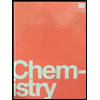# The following equation represents the oxidation of ammonia, NH3. 4NH3(g) + 5O2(g)  →  4NO(g) + 6H2O(g) At the same temperature and pressure, what is the maximum volume of nitrogen monoxide that can be obtained from 7.55 × 102 L of ammonia and 7.55 × 102 L of oxygen? a. 7.55 x 102L b. 3.36 x 102L c. 1.7 x 103L d. 1.51 x 103L e. 6.04 x 102L

Question
100%

The following equation represents the oxidation of ammonia, NH3.

4NH3(g) + 5O2(g)  →  4NO(g) + 6H2O(g)

At the same temperature and pressure, what is the maximum volume of nitrogen monoxide that can be obtained from 7.55 × 102 L of ammonia and 7.55 × 102 L of oxygen?

a. 7.55 x 102L

b. 3.36 x 102L

c. 1.7 x 103L

d. 1.51 x 103L

e. 6.04 x 102L

Expert Solution

### Want to see the full answer?

Check out a sample Q&A hereStudents who’ve seen this question also like:Chemistry by OpenStax (2015-05-04)
1st Edition
ISBN: 9781938168390
Author: Klaus Theopold, Richard H Langley, Paul Flowers, William R. Robinson, Mark Blaser
Publisher: OpenStax